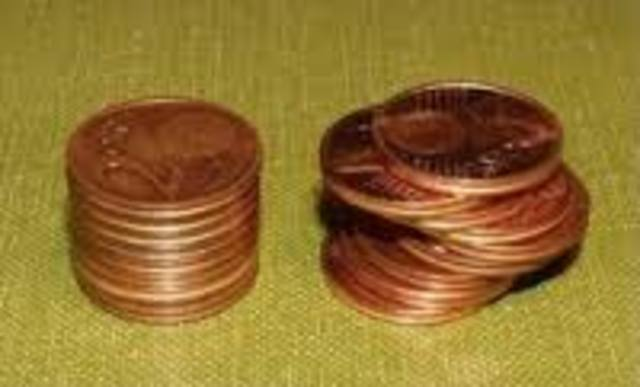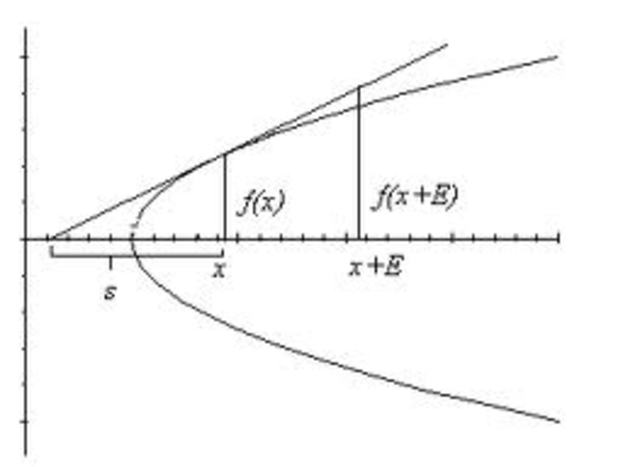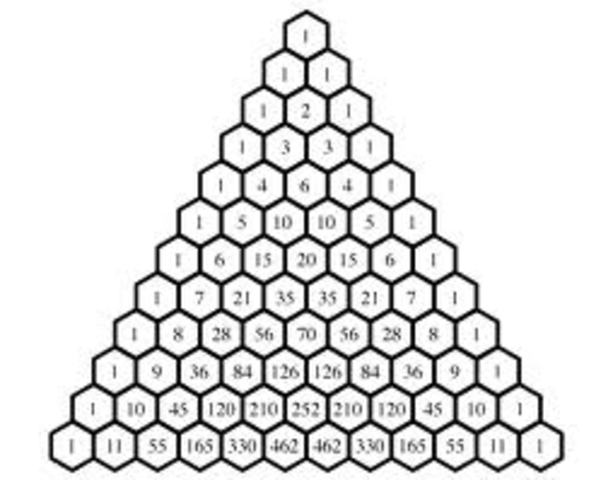# Newton, Leibniz and the Giants

• Period: Feb 15, 1564 to

## Galileo Galilei - Italy

The father of modern science and the last great Italian scientist before the rise of Cartesian geometry and then the Calculus of Newton and Leibniz. His death, followed by the untimely deaths of two of his top pupils (Cavalieri and Torricelli) in 1647 signaled the end of Italy as the center of European mathematical developments.
• Period: to

## Rene Descartes - France

Developer of Cartesian or analytic geometry. Descartes pioneered much of algebra's standard notation that is still in use today. Descartes was instrumental in the development of algebra becoming an accepterd means of mathematical reasoning. Before Descartes, Europeans had viewed geometry as a more fundamental or "pure" form of mathematics. His analytic, algebra based geometry served as an important base for the development of the calculus.
• Period: to

## Bonaventura Cavalieri - Italy

Cavalieri was a disciple of and frequent corresponder with Galileo. He was influential for his geometric methods of computing areas under curves. His work on area under curves paved the way for the integration
• Period: to

## Pierre de Fermat - France

Fermat developed a method for determining maxima, minima, and tangents to curves that was equivalent to modern day differentiation. Fermat also expanded on the work of Cavalieri by using geometric series to model the area under curves. Fermat published very little during his lifetime, but he was active in the mathematics community and his work was well known in his day.Fermat made great and lasting contributions in the fields of number theory and probability (with Pascal).
• Period: to

## John Wallis - England

An angry British mathematician known as much for his temper as he was for his mathematical contributions. His definition of an infinitesimal as being one divided by infinity served as a basis for his work in integral calculus. He is responsible for developing standard notation for rational powers. His work on furthering Cavalieri's theories was instrumental in the development of calculus. Wallis was involved in a number of bitter disputes and famously made many claims without substanial proof.
• Period: to

## Blaise Pascal - France

French mathematician most well known for his tabular notation of binomial coefficients. His work with Fermat helped develop the mathematical theory of probability. His work was particuluar influential to Leibniz who claimed it was paper by Pascal that caused him to have his biggest breakthrough with calculus. He abandoned mathematics after he had a religious experience in 1654.
• Period: to

## Isaac Barrow - England

Teacher of Newton and influential mathematician. Barrow improved upon Fermat's method of finding tangents and is credited with being one of the first to understand the inverse relationship between differential and integral calculus. He was a conservative mathematician who felt that geometry was the only proper form of math. This limited his ability to further his development of calculus.
• # Cavalieri's "Goemetria indivisibilibus..." is publishedCavalieri published his landmark geometric approach to integration which was built on the method of exhaustion. Cavalieri's work argued that volumes and areas should be computed as the sums of the voumes and areas of infitisimally thin cross sections. His resulting quadrature formula is the basis for the modern day inverse of the power rule that is used in integration. His work was influential to Wallis and Newton and to the development of calculus.
• # Descartes publishes La GeometrieDecartes published La Geometrie as an appendix to Discours de la methode. La Geometrie was the first work to propose the idea of combining algebra and geometry. Cartesian geometry (as it is often called) involves defining geometric shapes by algebraic equations and using these equations to manipulate these shapes or derive information about them. This concept would prove instrumental in influencing the work of Leibniz and Newton
• # Fermat's methods of finding maxima, minima, and tangents is first put into print.Fermat's methods had been around since the 1630's and had been circulated through mathematicians via letter writing. His method of finding maxima, minima, and tangents were essential to the work of Newton and Leibniz and was equivalent to differentiation. While most of Fermat's writings were published posthumously, this method was published in a book by Pierre Herigone in 1642.
• Period: to

## Isaac Newton - England

Newton is generally credited with being the first person to fully integrate and develop calculus practically. He used the ideas of rates of change and calculus to rewrite the rules of physics and optics. Newton's approach to calculus was very geometric and based upon real values which reflected the influences of Barrow and others on his work.
• Period: to

## Gottfried Leibniz - Germany

Leibniz was the first to publish a comprehensive work on calculus. While Newton developed his ideas earlier, Leibniz's calculus was the first to be published. Leibniz's approach to calculus was very different from Newton's. Leibniz's calculus was based on small, infinitesmal values and Leibniz created universal calculus notation that is still used today. He is credited (along with Newton) as being the discoverer of calculus.
• # Wallis publishes Arithmetica InfinitorumArithmetica Infinitorum synthesized the works of Descartes and Cavalieri by arithmetizing Cavalieri's painstaking process of primitive geometric integration. Wallis also introduces the symbol for infinity and develops the standard notation for powers, including rational exponents. Wallis is often criticized for questionable reasoning and proofs. In this book he makes bold, unproven claims that his integration can be applied to higher powers of x, fractional values of x and negative values.
• # Pascal publishes Treatise on the Sines of a Quadrant of a CirclePascal's work on the integration of a sine function was a huge influence on Leibniz and a precursor to calculus. Leibniz claims that it was while reading this work that he had a light burst upon him and he had his major breakthrough with calculus.
• Period: to

## Guillaume de l'Hopital - France

French Mathematician and regular corresponder with Leibniz and with the Bernoullis (amongst others). L'Hopital wrote the first textbook on the infitesmal calculus. He is also credited with (although he didn't invent) l'Hopital's rule for finding limits of indeterminate form.
• # Barrow publishes Lectiones geometriaeBarrow's lectures include both tangent problems and quadratures which were the most popular and cutting edge problems at the time. Barrow seemed to have an understanding that the problems were inversely related to each other and stood on the verge of a enormous breakthough, but his strict adherence to older geometry and his disdain for Cartesian mathematics limited his work and made his writings hard to understand.
• # Leibniz's Nova Methodus is publishedLeibniz publishes his first account of differential calculus. The title "Nova Methodus pro Maximus et Minimis, itemeque Tangentibus, qua nec Irrationales Quantitates Moratur (A New Method for Maxima and Minima, and Also for Tangents, which Is Not Obstructed by Irrational Quantities)" is an accurate description of what is in the book. In Nova Methodus, Leibniz introduces the product, quotient, and power rules. Leibniz is the first to use notations similar to what is still in use today.
• # Newton's Principia Mathematica is publishedNewton publishes his most famous work. While Principia is primarily a work about physics, the mathematics that he employs in creating his theories involves fluxions which was the Newtonian form of caculus. While the language of modern calculus is mostly absent from the book, many of his proofs involve a geometric form of calculus and explore concepts (rates of change, etc) that are essential elements of calculus.
• # l'Hopital publishes Analysis of the Infinitely Small to Understand Curvesl'Hopital's book is the first "textbook" on differential calculus. It is based upon the differential calculus of Leibniz. This book also marks the first appearance of L'Hopitals rule (which was actually created by Johann Bernoulli).
• # Newton's Method of Fluxions is publishedNewton's book (which was completed in 1671) is published posthumously. Fluxions is Newton's book on the subject of differential calculus. Even though it was not published until over 50 years after Leibniz's book, Newton had let it be known that he had already developed his calculus soon after Leibniz's book was published.